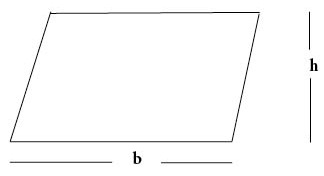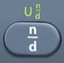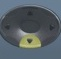## Parallelogram

Parallelogram = Flat Four-Sided Shape in which Opposite Sides are Parallel

#Basics
b = base
h = height
A = Area = area covering surface of parallelogram (measured in square units)

A rectangle is a special form of a parallelogram in which all angles are right angles.
A square is a special form of a parallelogram in which all angles are right angles and all sides are equal.

Formulas (Provided by Mathematics Formula Sheet)

A = bh

Formulas (NOT provided by Mathematics Formula Sheet)
b = A ÷ h
h = A ÷ b

Question
A solar panel in the shape of a parallelogram has a base of 6 feet and height of 3 feet.
What is its area?

18 square feet

A = bh
A = bh = 6 × 3 = 18

Calculator Click What You See Commentblinker clears screen
6 × 3 6*3 A = bh18 Answer
###### Parallelogram

Question
A solar panel in the shape of a parallelogram has a base of$\bf\displaystyle6\frac{1}{2}$ feet and height of$\bf\displaystyle3\frac{1}{4}$ feet.
What is its area in decimal form?

21.125 square feet

A = bh
A = bh =$\bf\displaystyle6\frac{1}{2}\times 3\frac{1}{4}$$\bf\displaystyle\frac{169}{8}$ = 21.125 after toggle

Calculator Click What You See Commentblinker clears screen612×314$\bf\displaystyle6\frac{1}{2}\times 3\frac{1}{4}$ A = bh$\bf\displaystyle\frac{169}{8}$21.125 Answer
(Toggle)
###### Parallelogram

Question
A solar panel in the shape of a parallelogram has an area of 96 square feet and a height of 8 feet.  What is its base?

12 feet

b = A ÷ h
b = 96 ÷ 8 = 12

Calculator Click What You See Commentblinker clears screen
96 ÷ 8 96÷8 b = A ÷ h12 Answer
###### Parallelogram

Question
A solar panel in the shape of a parallelogram has an area of 132 square feet and a base of 12 feet.  What is its height?

11 feet

h = A ÷ b
h = 132 ÷ 12 = 11

Calculator Click What You See Commentblinker clears screen
132 ÷ 12 132÷12 h = A ÷ b11 Answer
###### Parallelogram

Practice – Questions
1.  A window in the shape of a parallelogram has a base of 3 feet and height of 2 feet.
What is its area?

2.  A window in the shape of a parallelogram has a base of$\bf\displaystyle3\frac{1}{2}$ feet and height of$\bf\displaystyle2\frac{1}{4}$ feet.
What is its area in decimal form?

3.  A window in the shape of a parallelogram has a base of$\bf\displaystyle2\frac{3}{4}$ feet and height of$\bf\displaystyle1\frac{1}{4}$ feet.
What is its area in decimal form?

4.  A window in the shape of a parallelogram has an area of 156 square inches and a height of 12 inches.  What is its base?

5.  A window in the shape of a parallelogram has an area of 200 square inches and a base of 20 inches.  What is its height?